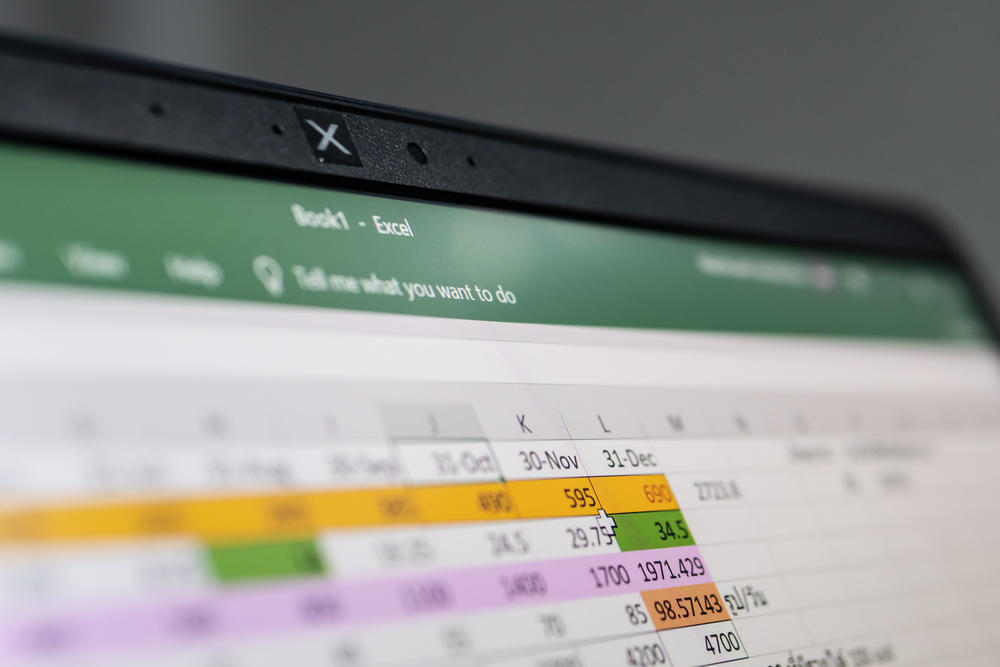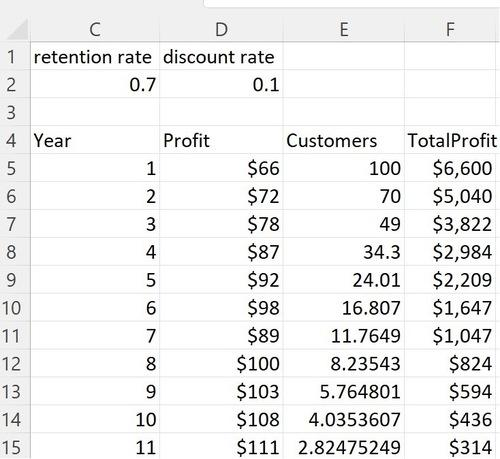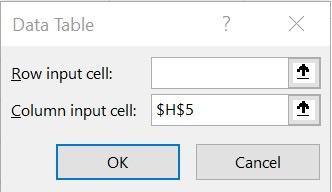# Calculating customer value using sensitivity analysis in ExcelCompanies need to accurately value their customers. After all, if it costs \$200 to acquire a customer who’s worth only \$150, then you will lose money on that customer. In this article we introduce a simple Excel model that is used to compute customer value. We’ll also share how to determine how sensitive our customer value estimate is to our estimated customer retention rate. Our work is shown in the workbook CalculateCustomerValue.xlsx.

Suppose we are trying to estimate the value of a bank’s credit card customer. From our data, we already know the average profit earned during each year the customer holds our credit card. To compute the customer’s value, we need to estimate how long the customer will use the credit card. To model how long a customer will use the card, we estimate an annual retention rate. For example, a retention rate of 0.7 means at the end of each year we keep 70% of our customers and lose 30% of our customers.

In this scenario, we assume we have a cohort of 100 customers and calculate our profit each year from remaining customers. Our model includes 50 years of profits. Column D in Figure 1 shows the profit from a single customer based on the length of time the customer has been with us. For example, during Year 1 we estimate a profit of \$66 per customer. During each successive year, profit levels increase each year until they level off at \$161 in years 19-50.

In Column E we compute the number of customers at the beginning of each year using the following formula:

(Customers beginning of year t+1) = (retention rate)*(customers beginning of year t.)

Copying the formula = E5*\$C\$2 from E6 to E7:E54 computes the number of customers remaining at the beginning of each year. For example, assuming a retention rate of 0.7, at the beginning of Year 2 we have 100*.70 customers.

Copying the formula = D5*E5 from F5 to F6:F54 computes our total profit for each year.Figure 1. Computation of annual profit.

Profit earned during later years is not as valuable as profit earned during earlier years. To account for this fact, we will discount future profits, assuming a discount rate of 10%, which implies that each dollar of Year 2 profit is worth 1/1.1 as much as a dollar of Year 1 profit. We can use Excel’s net present value (NPV) function to compute the total NPV (in Year 1 dollars) of 50 years of profit. The NPV function assumes the first cash flow in the selected range is one period from now. As shown in Figure 2, this requires us to compute the NPV per customer with the formula = (F5+NPV(D2,F6:F54))/100. This formula multiplies the Year 2 total profit, or \$5,040 by 1/1.1, the Year 3 total profit of \$3,822 by 1/1.12, and so on, to find that the value of each customer is \$209.49.  In reality, individual customers have different values, and sophisticated companies have the ability to accurately estimate the value of each customer.Figure 2. Total NPV per customer and sensitivity analysis on NPV.

## How to calculate customer retention rate through sensitivity analysis

Because we don’t know our retention rate, we want to conduct a sensitivity analysis, which tells us how sensitive customer value is to changes in the annual retention rate. A one-way data table will accomplish this goal. In a one-way data table, we can calculate how one or more outputs (in this case we have only a single output, customer NPV) depend on a single input (customer retention rate).

The one-way data table is shown in cell range I8:J16 of Figure 2. To create this data table, we enter the values of our input in I9:I16 and move up one row from the top of the inputs and one column to the right to cell J8:J16 and enter the formula (cell H5) that you want recalculated for each input value. Then select the table range (I8:J16) and select Data Table from the What-If Analysis icon. Then fill in the Data Table dialog box as shown in Figure 3.Figure 3. Data table settings.

These settings cause Excel to recompute cell H5 (NPV per customer) for retention rates between 0.1 and 0.95. The key takeaway is that a small increase in retention rate (say from 80% to 90%) can lead to a large (over 60%!) increase in customer value. As a result, in many situations, spending money on successful customer retention initiatives is worthwhile.

Keep strengthening your Microsoft Excel skills with a Becker CPE course! In “Buy or Rent; Lease or Buy?” Dr. Wayne Winston provides an overview of how to decide between buying or renting your residence, or buying or leasing your car, through Excel formulas.

### Now Leaving Becker.com

You are leaving the Becker.com website. Once you click “continue,” you will be brought to a third-party website. Please be aware, the privacy policy may differ on the third-party website. Adtalem Global Education is not responsible for the security, contents and accuracy of any information provided on the third-party website. Note that the website may still be a third-party website even the format is similar to the Becker.com website.